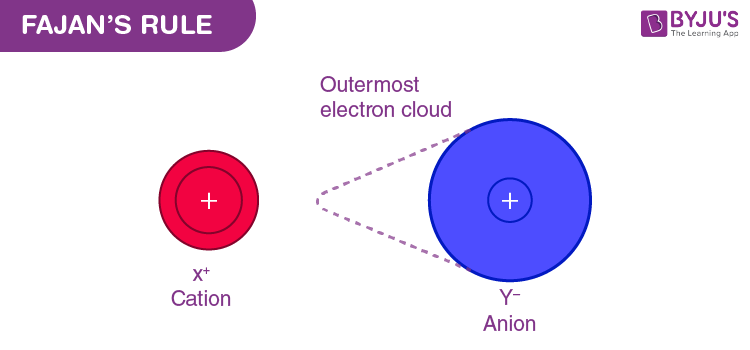Win up to 100% scholarship on Aakash BYJU'S JEE/NEET courses with ABNAT Win up to 100% scholarship on Aakash BYJU'S JEE/NEET courses with ABNAT

# Fajan’s Rule

The Fajans’ rule predicts whether a chemical bond will be covalent or ionic. A few ionic bonds have partial covalent characteristics, which were first discussed by Kazimierz Fajans in 1923. He was able to predict ionic or covalent bonding with attributes like ionic and atomic radius with the help of X-ray crystallography.

## What Is the Fajans’ Rule?

We classify certain compounds as ionic and other compounds as covalent. Among the alkali chlorides, which is the most ionic? To answer these kinds of questions, we employ the Fajans’ rules as a tool.Fajans’ Rule for Ionic and Covalent Compounds

To understand the Fajans’ rule, let us first understand a few terms:

### Polarising power

It is the extent to which a cation can polarise an anion. It is proportional to charge density. Charge density is the ratio of charge to volume. Polarising power α Charge density. The more the charge density, the greater the polarising power for that cation.

### Polarisability

It is the extent to which an ion can be polarised. It can also be defined as the ease with which an ion can be polarised. Polarisation is the distortion of a spherically symmetric electron cloud to an unsymmetric cloud.

## Postulates of Fajans’ Rule

The rule can be stated on the basis of 3 factors, and they are:

1. Size of the ion: Smaller the size of the cation, the larger the size of the anion and the greater the covalent character of the ionic bond.
2. The charge of cation: Greater the charge of the cation, the greater the covalent character of the ionic bond.
3. Electronic configuration: For cations with the same charge and size, the one with (n-1)dnso which is found in transition elements, has greater covalent character than the cation with nsnp6 electronic configuration, which is commonly found in alkali or alkaline earth metals.

## Explanation of Fazans’ Rule

Rule 1:

The first rule speaks about the polarising power of the cation. If the cation is smaller, then we can say that the volume of the ion is less. If the volume is less, we can conclude that the charge density of the ion would be high.

Since the charge density is high, the polarising power of the ion would be high. This makes the compound to be more covalent.

Rule 2:

The second rule speaks about the polarizability of the anion. The larger the anion, the less the effective nuclear charge that holds the valence electron of the ion in place. Since the last electron is loosely bound in large anions, it can easily be polarised by a cation, thereby making the compound more covalent.

Rule 3:

The third rule is a special case. Let us use an example to explain this point.

Example: If we want to find the more covalent compound among HgCl2 and Calcium Chloride, we cannot use size as a factor to conclude. This is because both Hg2+ and Ca2+ are of almost equal size. To explain this, we employ the third rule.

The electronic configuration of Hg2+ is 6s0 5d10. This configuration is called pseudo-octet because the d-orbital is fully filled, but the element does not have 8 electrons or an octet.

We know that d orbitals are not good at shielding, so we can say that the anion (Cl) would be more polarised because the d orbital is poor at shielding, making HgCl2 more covalent than CaCl2 because Ca2+ ion has a noble gas configuration.

Now, to answer the question that we asked first, among the alkali chlorides, which one is the most covalent:

Since the anion is the same, we have to compare the cations. According to Fajans’ rules, the smaller the cation, the more the covalency. Therefore, LiCl is the most covalent.

### Let Us Understand Fajans’ Rule Using a Detailed Illustration

#### Consider Aluminium Iodide (AlI3)

This is an ionic bond which was formed by the transfer of electrons.

• The iodine being bigger, has a lesser effective nuclear charge. Thus, the bonding electrons are attracted lesser towards the Iodine nucleus.
• On the contrary, the aluminium having three positive charges attracts the shared pair of electrons towards itself.
• This leads to insufficient charge separation for it to be ionic, and so it results in the development of a covalent character in AlI3.

#### Consider Aluminium Fluoride (AlF3)

This is an ionic bond which was also formed by the transfer of electrons. But here, the fluorine being smaller attracts the shared pair of an electron more towards itself, and so there is sufficient charge separation to make it ionic.

## Examples of Fajans’ Rule

Illustration 1: Which compound should theoretically be the most ionic and the most covalent among the metal halides?

Solution:

The smallest metal ion and the largest anion should technically be the most covalent.

Therefore, LiI is the most covalent.

The largest cation and the smallest anion should be the most ionic. Therefore, CsF should be the most ionic.

Illustration 2: Arrange the following according to the increasing order of covalency:

• NaF, NaCl, NaBr, NaI
• LiF, NaF, KF, RbF, CsF

Solution:

1. Since the cation is the same, compare the anions. Amongst the anions, the larger the size would be the covalency. Therefore, the order is: NaF < NaCl < NaBr < NaI

2. Here, the anion is the same, so we compare it with cations. The smaller the cation, the more the covalency. Therefore, the order is: CsF < RbF < KF < NaF < LiF

### Fajans’ rule can be summarised as follows:

 Ionic Characteristics Covalent Characteristics Large Cation Small Cation Small Anion Large Anion Small Charge Large Charge

Try the following:

1. CuCl is more covalent than NaCl. Why?
2. LiCl is more soluble in pyridine than in H2O. Why? [Hint: Apply Fajans’ Rule]
Test your knowledge on Fajans Rule